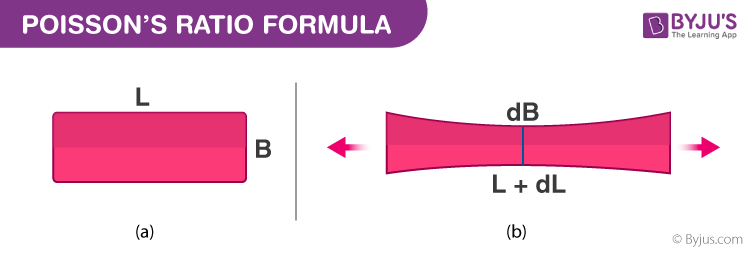# Poisson's Ratio - Longitudinal Strain and Lateral Strain

In mechanics, Poisson’s ratio is the negative of the ratio of transverse strain to lateral or axial strain. It is named after Siméon Poisson and is denoted by the Greek letter ‘nu’, It is the ratio of the amount of transversal expansion to the amount of axial compression for small values of these changes.

## What Is Poisson’s Ratio?

Poisson’s ratio is “the ratio of transverse contraction strain to longitudinal extension strain in the direction of the stretching force.” Here,

• Compressive deformation is considered negative
• Tensile deformation is considered positive.
 Symbol Greek letter ‘nu’,ν Formula Poisson’s ratio = – Lateral strain / Longitudinal strain Range -1.0 to +0.5 Units Unitless quantity Scalar / Vector Scalar quantity

## Poisson’s Ratio Formula

Imagine a piece of rubber, in the usual shape of a cuboid. Then imagine pulling it along the sides. What happens now?It will compress in the middle. If the original length and breadth of the rubber are taken as L and B respectively, then when pulled longitudinally, it tends to get compressed laterally. In simple words, length has increased by an amount dL and the breadth has increased by an amount dB.

In this case,

$$\begin{array}{l}\varepsilon _{t}=-\frac{dB}{B}\end{array}$$
$$\begin{array}{l}\varepsilon _{l}=-\frac{dL}{L}\end{array}$$

The formula for Poisson’s ratio is,

$$\begin{array}{l}Poisson’s\;ratio=\frac{Transverse\;strain}{Longitudinal\;strain}\end{array}$$
$$\begin{array}{l}\Rightarrow \nu =-\frac{\varepsilon _{t}}{\varepsilon _{l}}\end{array}$$

where,

εt is the Lateral or Transverse Strain

εl is the Longitudinal or Axial Strain

ν is Poisson’s Ratio

The strain on its own is defined as the change in dimension (length, breadth, area…) divided by the original dimension.

### Poisson’s Effect

When a material is stretched in one direction, it tends to compress in the direction perpendicular to that of force application and vice versa. The measure of this phenomenon is given in terms of Poisson’s ratio. For example, a rubber band tends to become thinner when stretched.

## Poisson’s Ratio Values for Different Material

It is the ratio of transverse contraction strain to longitudinal extension strain in the direction of the stretching force. There can be a stress and strain relation that is generated with the application of force on a body.

• For tensile deformation, Poisson’s ratio is positive.
• For compressive deformation, it is negative.

Here, the negative Poisson ratio suggests that the material will exhibit a positive strain in the transverse direction, even though the longitudinal strain is positive as well.

For most materials, the value of Poisson’s ratio lies in the range, 0 to 0.5.

A few examples of poisson’s ratio are given below for different materials.

 Material Values Concrete 0.1 – 0.2 Cast iron 0.21 – 0.26 Steel 0.27 – 0.30 Rubber 0.4999 Gold 0.42 – 0.44 Glass 0.18 – 0.3 Cork 0.0 Copper 0.33 Clay 0.30 – 0.45 Stainless steel 0.30 – 0.31 Foam 0.10 – 0.50

## Watch the video below to understand the Poisson’s ratio in detailPhysics Related Topics:

## Frequently Asked Questions – FAQs

Q1

### Does Poisson’s ratio depend on temperature?

In general, Colder temperature decreases both strains and high-temperature increases both horizontal and vertical strain. Thus, the net effect on Poisson’s Ratio is small since the change in both horizontal and vertical strain is by a similar amount.
Q2

### Is Poisson’s ratio constant?

Poisson’s ratio for material remains approximately constant within elastic limits.

Q3

### Define Poisson’s ratio.

The ratio of transverse strain to longitudinal strain in the direction of the stretching force.
Q4

### Write the Poisson’s ratio formula.

$$\begin{array}{l}Poisson’s\;ratio=\frac{Transverse\;strain}{Longitudinal\;strain}\end{array}$$
Q5

### State true or False: Poisson’s ratio is negative for Tensile deformation.

False. Poisson’s ratio is Positive for Tensile deformation
Q6

### What is Poisson’s ratio of concrete?

The Poisson’s ratio of concrete is 0.1 to 0.2.
Q7

### What does the Poisson’s ratio 0.5 mean?

Poisson’s ratio 0.5 means a perfectly in-compressible material is deformed elastically at small strains.

Q8

### What are the units of Poisson’s ratio?

Poisson’s ratio is the unitless scalar quantity.
Q9

### What is Poisson’s ratio of cork?

Poisson’s ratio of cork is 0.0.

Hope you have understood Poisson’s ratio, how it is defined, its symbol, units, formula, terms and values for various materials. Stay tuned with BYJU’S for more such interesting articles. Also, register to “BYJU’S-The Learning App” for loads of interactive, engaging physics-related videos and unlimited academic assistance.

Test your Knowledge on Poissons Ratio